# 1736 Fun With Magic Squares

Contents

### Today’s Puzzle is a 7×7 Magic Square:

1736 is the sum of seven consecutive numbers:
245 + 246 + 247 + 248 + 249 + 250 + 251 = 1736.

That means 1736 is the magic sum of a 7×7 Magic Square with the numbers from that sum running along the diagonal. Can you write the rest of the consecutive numbers from 224 to 272 to complete the magic square?If you need some help, trace with your finger while you count from 224 to 272 and see where the numbers fall diagonally on this completed magic square. You should notice that you always place the next number diagonally above to the right unless you can’t, in which case you place the next number directly under your last entry. Sometimes you will have to leave the square and get back on it on the opposite side of the square to maintain the diagonal.

After studying the pattern, try to do it yourself. This excel sheet has a place for you to type the numbers for this magic square and every other magic square discussed in this post. The spreadsheet will even keep a running sum of each column, row, and diagonal as you type in the numbers: 12 Factors 1730-1738.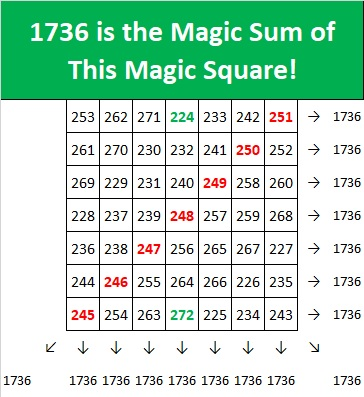The current year is also divisible by seven, and consequently
286 + 287 + 288 + 289 + 290 + 291 + 292 = 2023.
Can you make a 7×7 Magic Square with 2023 as the magic sum?Here is that completed square as well. It follows the same mostly diagonal path as the previous completed magic square:### 17×17 Magic Square:

2023 is divisible by 17, and consequently

111 + 112 + 113 + 114 + 115 + 116 + 117 + 118 + 119 + 120 + 121 + 122 + 123 + 124 + 125 + 126 + 127 = 2023.

All that means that 2023 is also the magic sum of a 17×17 Magic Square, but we also have to use some negative numbers to make it. Trace the numbers beginning with -25 and notice the same diagonal pattern in this magic square.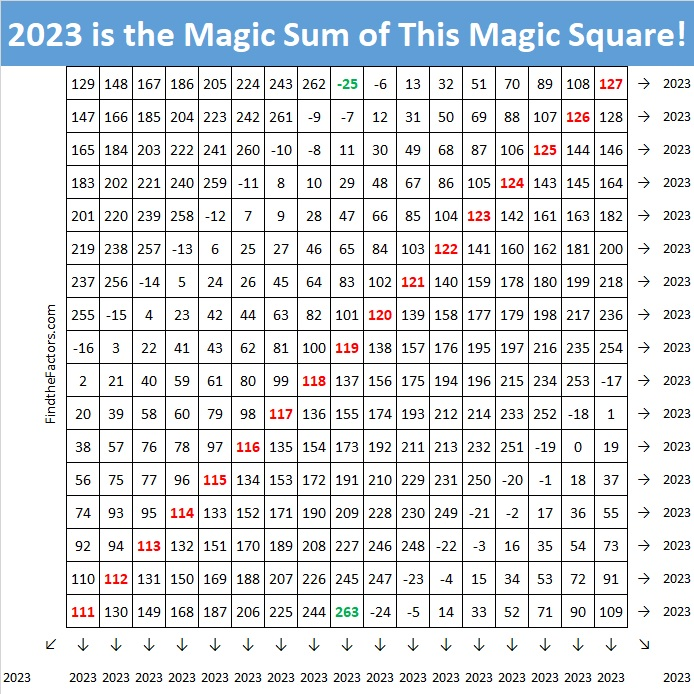### 14×14 Magic Square:

It’s a bit trickier, but since 2023 is divisible by seven but not by 14, it is also the magic sum of a 14×14 Magic Square. To make it start off by dividing the 14×14 square into four 7×7 squares. Place the numbers from 47 to 95 in the top left square, the numbers from 96 to 144 in the bottom right square, the numbers from 145 to 193 in the top right square, and the numbers from 194 to 242 in the bottom left square.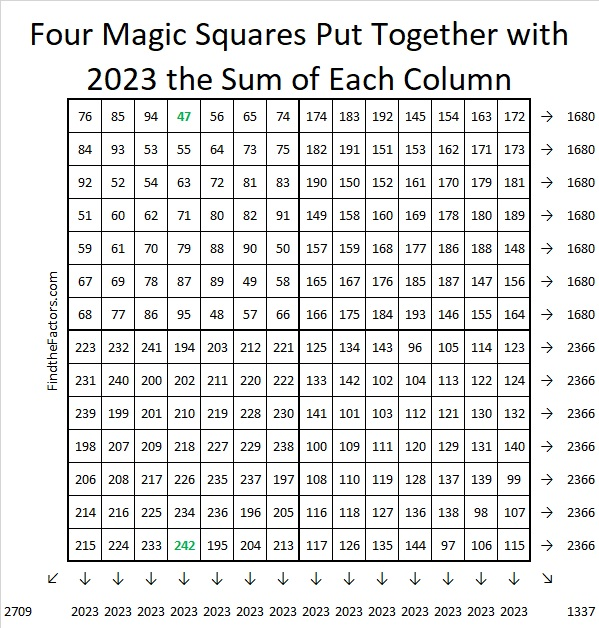Notice that all of the columns show our desired magic sum, but none of the rows or diagonals do. We need to switch some of the numbers to fix that. Switch the shaded areas indicated below with their corresponding darker shaded areas: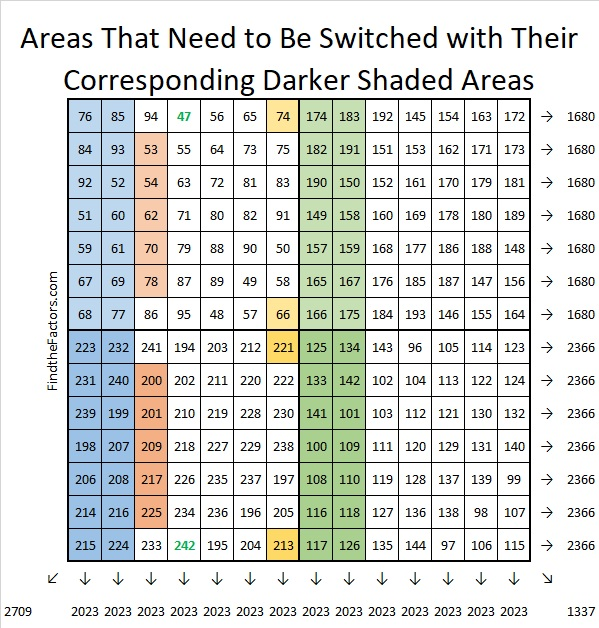And you will successfully create this beautiful magic square where every row, column, and diagonal equals 2023.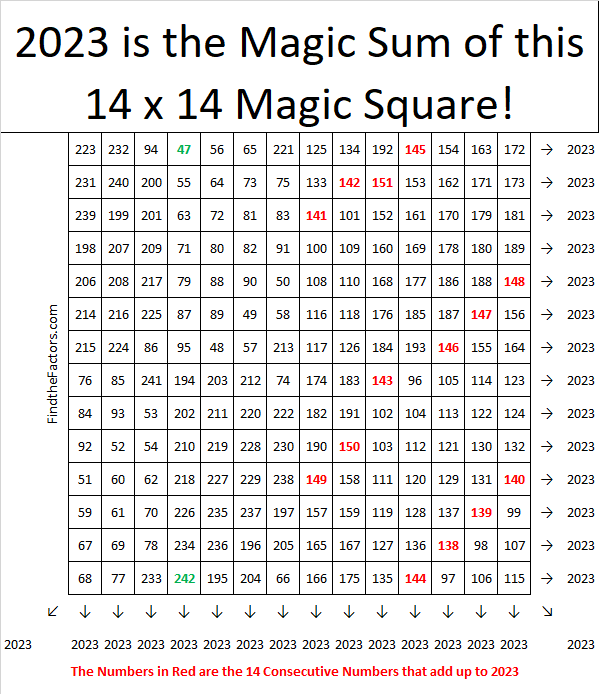Notice that the 14 consecutive numbers that add up to 2023 are all over the square.
138+139+140+141+142+143+144+145+146+147+148+149+150+151 = 2023

Any other magic square for 2023 would be too big and have so many negative numbers.

### 16×16 Magic Square:

1736 is divisible by 8, but not by 16, so there are 16 consecutive numbers that add up to 1736:

101 + 102 + 103 + 104 + 105 + 106 + 107 + 108 + 109 + 110 + 111 + 112 + 113 + 114 + 115 + 116 + 117 = 1736.

Making a 16×16 Magic Square will be a bit tedious, but so satisfying. Start by placing the numbers from -19 to 236 in order from left to right filling in each 4×4 square before moving onto the next 4×4 square, as illustrated below: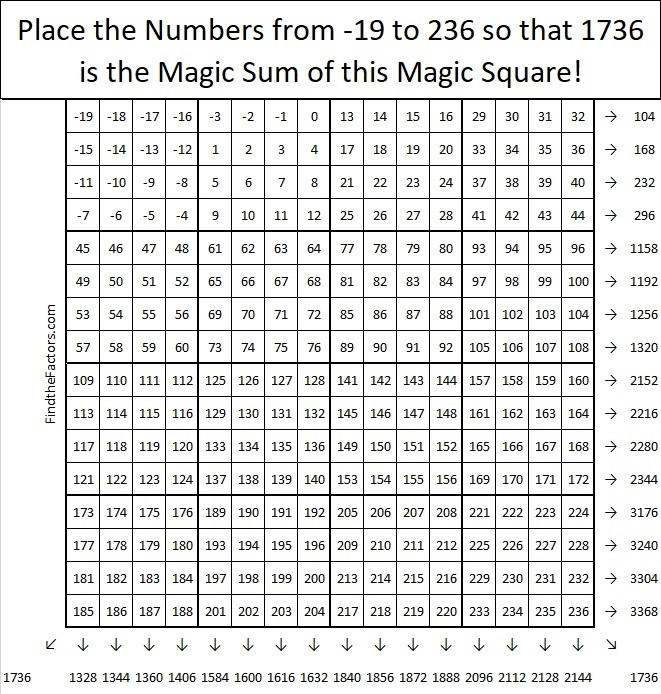Notice that only the diagonals show the desired sum of 1736.

Next, we want to flip the diagonals of each small 4×4 square, as shown below.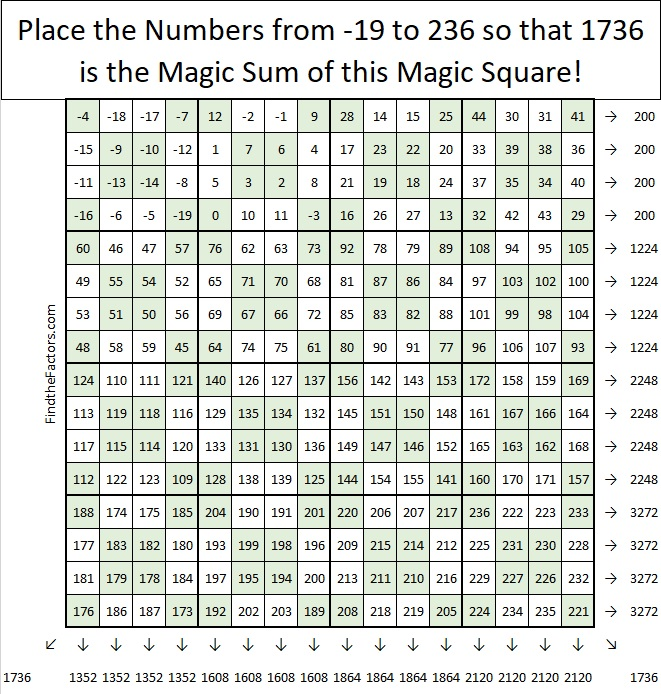The diagonals still have the correct sum, and look at those sets of four columns or four rows that have equal sums! Now think of the whole square as one big 4×4 Magic Square, and flip its diagonals as shown below:Oh, it is a thing of beauty, don’t you agree?

### Factors of 1736:

• 1736 is a composite number.
• Prime factorization: 1736 = 2 × 2 × 2 × 7 × 31, which can be written 1736 = 2³ × 7 × 31.
• 1736 has at least one exponent greater than 1 in its prime factorization so √1736 can be simplified. Taking the factor pair from the factor pair table below with the largest square number factor, we get √1736 = (√4)(√434) = 2√434.
• The exponents in the prime factorization are 3,1 and 1. Adding one to each exponent and multiplying we get (3 + 1)(1 + 1) (1 + 1) = 4 × 2 × 2 = 16. Therefore 1736 has exactly 16 factors.
• The factors of 1736 are outlined with their factor pair partners in the graphic below.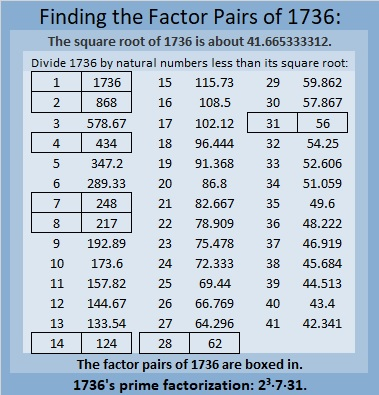### More About the Number 1736:

1736 is the difference of two squares in four different ways:
435² – 433² = 1736,
219² – 215² = 1736,
69² – 55² = 1736, and
45² – 17² = 1736.

This site uses Akismet to reduce spam. Learn how your comment data is processed.